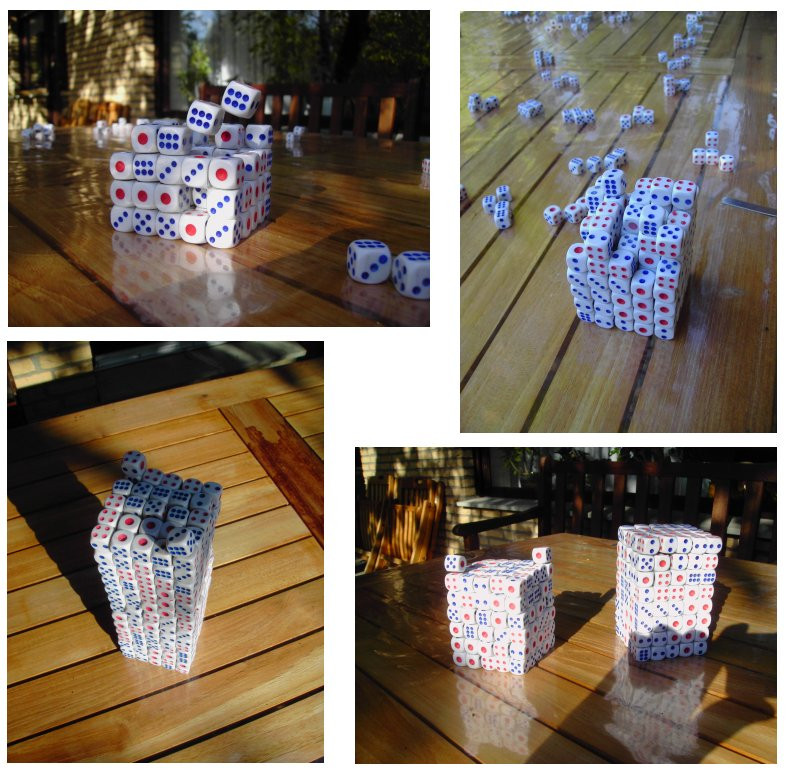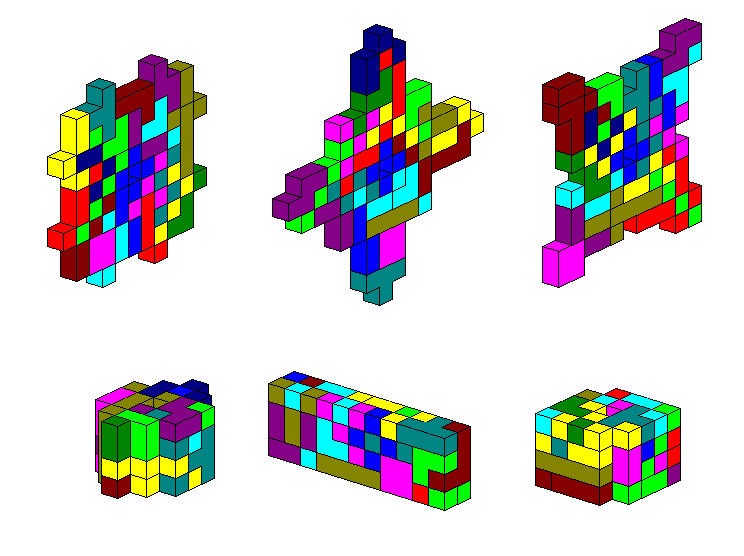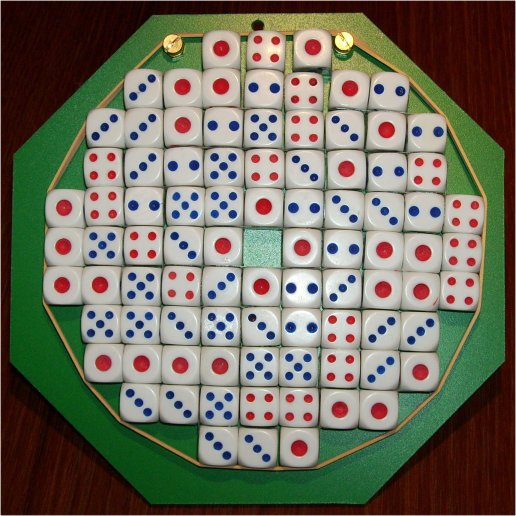# Pseudo-Tetracubes:

### 3-dim Pseudo-Tetracubes:

There are 88 pseudo-tetracubes with a total volume of 352 cubes. Under checkerboard coloring we have an odd number of unbalanced pieces and the total numbers of black and white cubes are odd. In a box with 352 cubes at least one edge is even and we have 176 white and 176 black cubes. Therefore a construction of a box is impossible.After the computer had found a solution for a 5x5x14 tower with two single cubes on the top I decided to check the solution in a real construction, because I couldn't rule out problems while putting the pieces together. Here are the real pieces.With the flexible stripes connecting the cubes it was no problem to finish the tower.Here are the
layers.

### 2-dim Pseudo-Tetracubes:

If you only use the 22 flat pieces you can get some nice symmetric figures. If the figure is flat the hole in the X-piece cannot be filled since all adjacent edges are joined. In three dimension constructions without holes are possible as shown by the 2x4x11 box.The solutions are
here.

One construction is shown where the pieces are fixed by an elastic band. So I can dispay it in vertical position.Index
Home